\$B:#>,\$N0lGU(B

\$BKhF|!":#>,\$N0lGU\$r>e\$2\$F\$\$\$^\$9!#(B
\$B:#>,0{\$`\$b\$N\$K:\$\$C\$?\$i!";29M\$K\$7\$F\$_\$F\$/\$@\$5\$\$!#(B

2023\$BG/(B9\$B7n(B

 1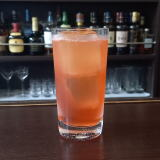(I7^L^1/L_%;O0(B 2(I;^%V:JO(B 3 \$B5Y\$_(B 4(ID^W:^]LW2(B 5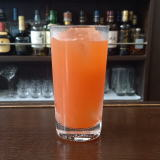(IJ^0F]8^%;](B 6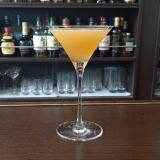(IC^(7%C^(7(B 7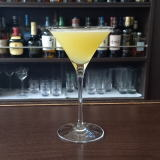(IJ_0Q%K^0A(B 8(IZ2F0%L^Y0(B 9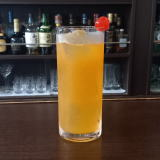(IT0%T0(B 10 \$B5Y\$_(B 11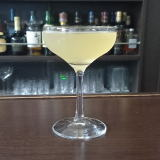(I@]6Z0%2]M_X1Y(B 12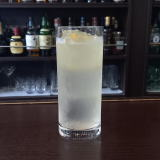(IK_0A%BX0%L(=^(B 13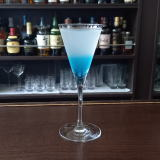(I50<,]%L^Y0(B 14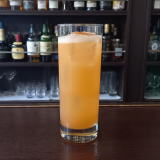(IS]:^X1](B 15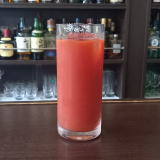(IL^W/C^(%R1X0(B 16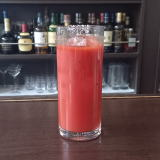(IJ^0<^]%R1X0(B 17 \$B5Y\$_(B 18\$B5Y\$_(B 19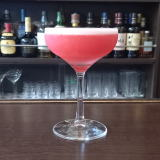(I>L_C]J^0%S0](B 20(I1J^]A-0Y(B 21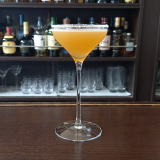(I8W 22(ILZ]A%ZN^X-0<.](B 23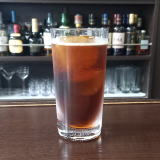(IWQ%:08(B 24 \$B5Y\$_(B 25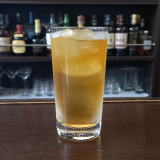(IZ9^4%J_]A(B 26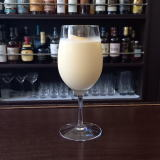(I1D^3^+60D%PY8(B 27(I=@0(B 28(I;]>/D%D^W2J^0(B 29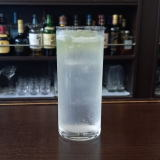(I=62%N^0Y(B 30 \$B5Y\$_(B